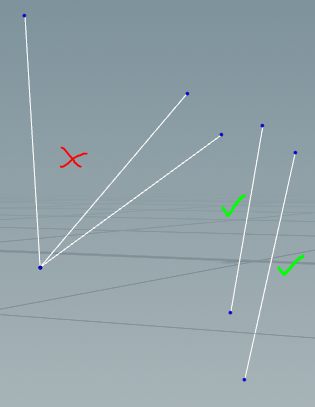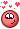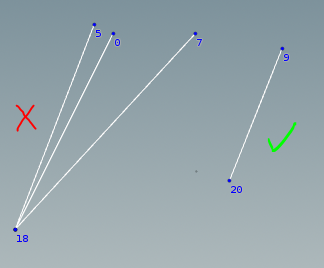## Recommended Posts

Hi,

I'm trying to create polylines between two groups of points, but not use the same 'destination' point twice.

The points are divided into two groups (upper and lower) and I'm using a point wrangle (with Group set to 'upper') to create the polylines:

```int nrpt = nearpoint(0, 'lower', @P);
setpointgroup(0, 'lower', nrpt, 0);```

By removing the destination point (nrpt) from the 'lower' point group I was hoping that nearpoint() wouldn't return that point again. It creates the polylines and removes the destination point from the point group, but it still uses that point again in the next iteration.

I thought this might be because the wrangle is multi-threaded and so the call to setpointgroup() is too late (the other threads have already done their thing) so I tried using a Detail wrangle instead:

```int npts = npoints(0);

for(int i = 0; i < npts; i++) {
if (inpointgroup(0, 'upper', i))
{
int nrpt = nearpoint(0, 'lower', point(0, 'P', i));
setpointgroup(0, 'lower', nrpt, 0);
}
}```

Unfortunately I get the same resultAnyone got any ideas?

test .hip attached...

polyline_connect.hipnc##### Share on other sites

Just play with 1 inp and 2 inp on Wrangle ( code found here on Odforce).```int drawLine(vector f; vector t){
return line;
}
//-----------------------------

if(npoints(1)>0){
}

int maxLines=chi("maxLineCount");
float minLen=chf("minLineLength");

int randomConnect=chi("randomConnect");
int keepPointCount=min(1, max(0,chi("keepPointCount")));
int runner=0;
vector curPos;
int pt;
if(randomConnect == 0){
for(int x=0; x<len(pts);++x){
pt=pts[x];

if(runner > maxLines){ break; }

if(keepPointCount){
int to=pt;
}
}else{
drawLine(v@P,curPos);
}
runner++;
}
}
}else{
int l=len(pts);
float fl=float(l);
int rander=pts[int(random(@ptnum*fl+randomConnect)*fl*fl) % l];
if(keepPointCount){
int to=rander;
}
}else{
drawLine(v@P,curPos);
}
}```

##### Share on other sites

Thanks @Librarian, but this seems to give me the same resultsFor each point in the upper group, I want to draw a line to the nearest point in the lower group that doesn't already have a line connecting it.

I'm probably missing something, but I can't see an 'already connected' check in the code you posted?##### Share on other sites

Fixed it!

In a Detail wrangle:

```int npts = npoints(0);
int connected[];
//for each point
for(int i = 0; i < npts; i++) {
if (inpointgroup(0, 'upper', i))
{
//find all nearest lower pts
int nrpts[] = nearpoints(0, 'lower', point(0, 'P', i), 1000, 1000);

//loop through lower points
for(int j = 0; j < len(nrpts); j++) {
if(connected[nrpts[j]] != 1){
connected[nrpts[j]]=1;
break;
}
}
}
}```

By creating a new array to store the 'connectedness' of each point I could remove the setpointgroup() logic.

Makes sense now I think about it - the wrangle was pulling in the nearpoints (and their group data) from upstream each iteration!

## Create an account

Register a new account